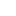# Determination of heat of solution by Calorimetric method

#### Heat of solution

The heat of the solution of a solid or liquid solute or substance can be determined in practically the same manner as that can be used for the determination of the heat of neutralization. The heat which evolves or absorbed on dissolving a substance depends on the amount of water or other solvent used, the statement of the heat of solution has a definite meaning only when the concentration of solution formed is well known. It depends on the dilution of the solution. When dilution is so great that further dilution is unaccompanied by any heating effect, then the heat measured per mole of solute is known as the heat of solution at infinite dilution.

#### Calorimetry:

Calorimetry is the measurement of the heat transfer into or out of a system during a chemical reaction or a physical process taking place under consideration.

A calorimeter is an insulated container that is used to measure the changes in heat taking place during a chemical reaction. Most of the reactions that can be analyzed under the Calorimetric experiment are either liquid or aqueous solutions. A typically used and inexpensive calorimeter consists of

• A set of nested foam cups
• A lid to limit the heat of exchange between the liquid in the cup and
• The air in the surrounding

In a typical Calorimetric experiment, known volumes of reactants are placed into separate containers or beakers and the temperature of each one is measured separately. They are then mixed into the calorimeter, which starts the reaction. The reactant mixture is stirred until the reaction is completer while the temperature of the reaction is continuously monitored.

#### Apparatus:

• Calorimeter
• Beaker
• Thermometer
• Tripod-stand with gauze

#### Procedure:

1. First of all the calorimeter was taken and washed properly and after drying empty calorimeter was weighed. Added about 50ml of cold water and reweight of cold water(W1 )
2. Then with the help of a thermometer temperature of cold water was measured at the time (let this time was zero) and check the temperature after every 30 seconds and 7 readings were taken the was T­2.
3. At the same time, a cleaned beaker was taken and added 50ml of water into it, and was heated up to 70C0. After reaching on the 70Co, the burner was removed and allow it to cool and noted the temperature changes after every 30 seconds and taken 5 reading. This was the temperature of hot water (T1).
4. Now beaker with water was weighed to measure (W­2).
5. Then quickly this warm water was added to the calorimeter in which cold water was already present. By this, a mixture of cold and hot water was formed.
6. Then started to note temperature changes in the mixture after 30 seconds by vigorous stirring. After few minutes the temperature of the mixture becomes constant and no further changes occurred. The measured temperature was the temperature of mixture (T)
7. Then calculated the heat capacity of the calorimeter by using the formula

CPx=CP1W1∆T – CP2W2∆T/∆T2

#### Calculations and observation:

 Time (sec) Temp. of cold water T2 (Co) Temp. of Hot water T1 (Co) Temp. of mixture T (Co) 0 24 70 30 24 41 60 24 40.5 90 24 40 120 24 40 150 41 180 40.5 210 40 240 40

W1= filled colorimeter – empty

=135.03-85.86=49.17g

W2 = filled beaker – empty

= 97.4 – 49.27 = 48.47g

68-40 = 28

= 40 -24 =16

CPx=CP1W1∆T – CP2W2∆T/∆T2

= 1(49.17)(28) – 1(48.47)(16)/16

= 601.26 /16

#### Result:

The specific heat capacity of the calorimeter is 37.57 J/K

#### Procedure:

• First of all the required glassware was washed and dried properly and accurately
• The weight of the empty calorimeter was weighed with the help of an electrical balance
• 5o ml of the water was taken in the calorimeter and noted the temperature with the help of thermometer with constant stirring after every 30 seconds
• Then 0.5 g of NaCl was weighed and added to the calorimeter
• The change in temperature was noted after every 30 seconds with constant stirring
• When temperature change became constant, the stopped the stirring and weight of solution was weighed
• The molality of NaCl was calculated
• The enthalpy of NaCl solution was calculated by using the formula

∆Hn = WCPx × ∆T + Cp∆T / m

#### Observations and Calculation:

 Time seconds Temp. of cold water Temp. of solution 0 21 30 21 60 21 90 21 120 21 150 21 180 21 210 20.8 240 20.6 270 20.5 300 20.5

As

∆T = Tcold – Tsolution

= 21 – 20.5

= 0.5 oC

Weight of empty calorimeter = 88.44 g

Weight of filled calorimeter = 137.65 g

Weight of solution = 49.21 g

As we know

CPx = 37.57 / K

Molality= mass of solute × 1000 /molar mass ×

Weight of solution

= 0.5 × 1000 / 58.5 × 49.21

= 0.17 mol /g

∆Hn = WCPx × ∆T + Cp∆T / m

= 49.21(37.57) (0.5) + 1(0.5) / 0.17

∆Hn = 5440.5 KJ/mol

#### Result:

The enthalpy of NaCl solution is 5440.5 KJ/mol

#### Errors and precautions:

During the performing of the experiment, a no of systematic and individual errors may occur such as systematic error and random errors. These errors may alter or disturb the result of our experiment. Determination errors may be minimized by using a different techniques and also by extensive care and by finding fault in concentration.